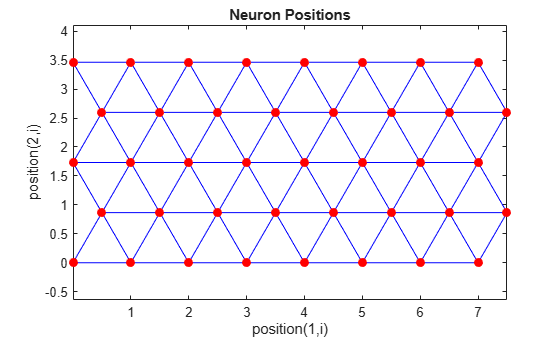# hextop

Hexagonal layer topology function

## Syntax

```hextop(dimensions) ```

## Description

`hextop` calculates the neuron positions for layers whose neurons are arranged in an `N`-dimensional hexagonal pattern.

`hextop(dimensions)` takes one argument:

 `dimensions` Row vector of dimension sizes

and returns an `N`-by-`S` matrix of `N` coordinate vectors where `N` is the number of dimensions and `S` is the product of `dimensions`.

## Examples

collapse all

This example shows how to display a two-dimensional layer with 40 neurons arranged in an 8-by-5 hexagonal pattern.

```pos = hextop([8 5]); plotsom(pos)```## Version History

Introduced before R2006a